Tutorials
python

# if…elif…else in Python

if…elif…else are conditional statements that provide you with the decision making that is required when you want to execute code based on a particular condition.

The if…elif…else statement used in Python helps automate that decision making process.

## if condition

The if condition is considered the simplest of the three and makes a decision based on whether the condition is true or not. If the condition is true, it prints out the indented expression. If the condition is false, it skips printing the indented expression.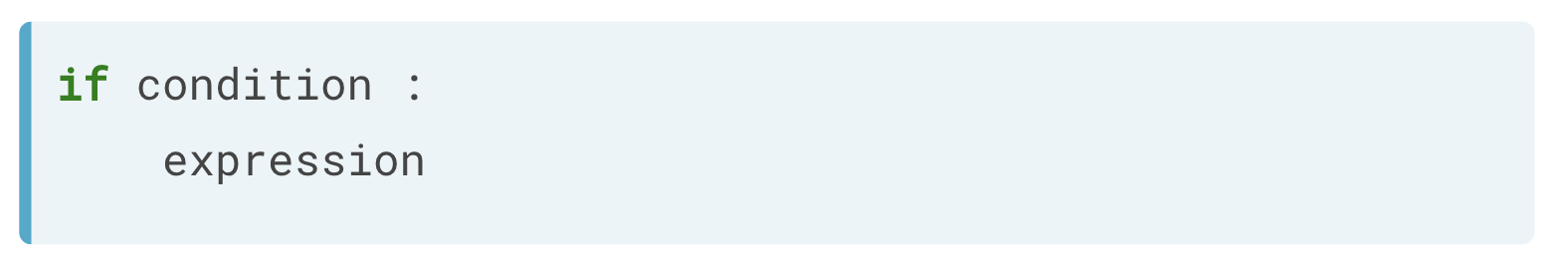### Example of if

Suppose you have a variable z, equal to 4. If the value is 'even', you will print z is 'even'. You will use modulo operator 2, which will return 0 if z is 'even'. As soon as you run the below code, Python will check if the condition holds. If True, the corresponding code will be executed.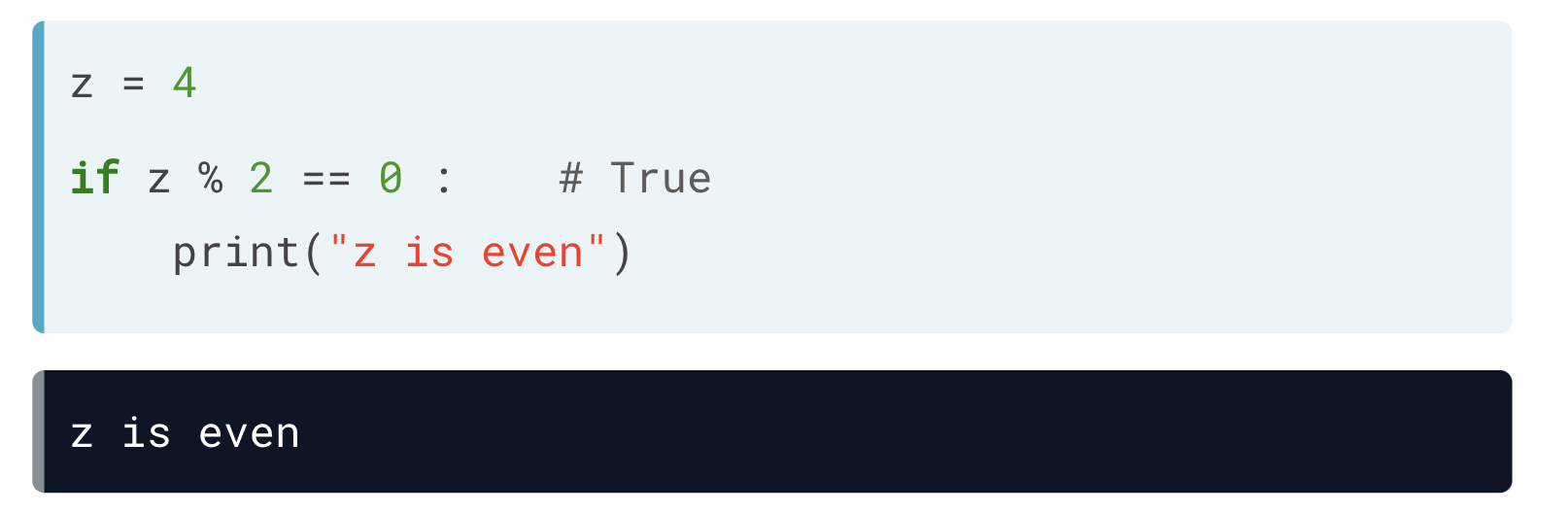##### Example of multiple lines inside if statement

It is perfectly fine to have more lines inside the if statement, as shown in the below example. The script will return two lines when you run it. If the condition is not passed, the expression is not executed.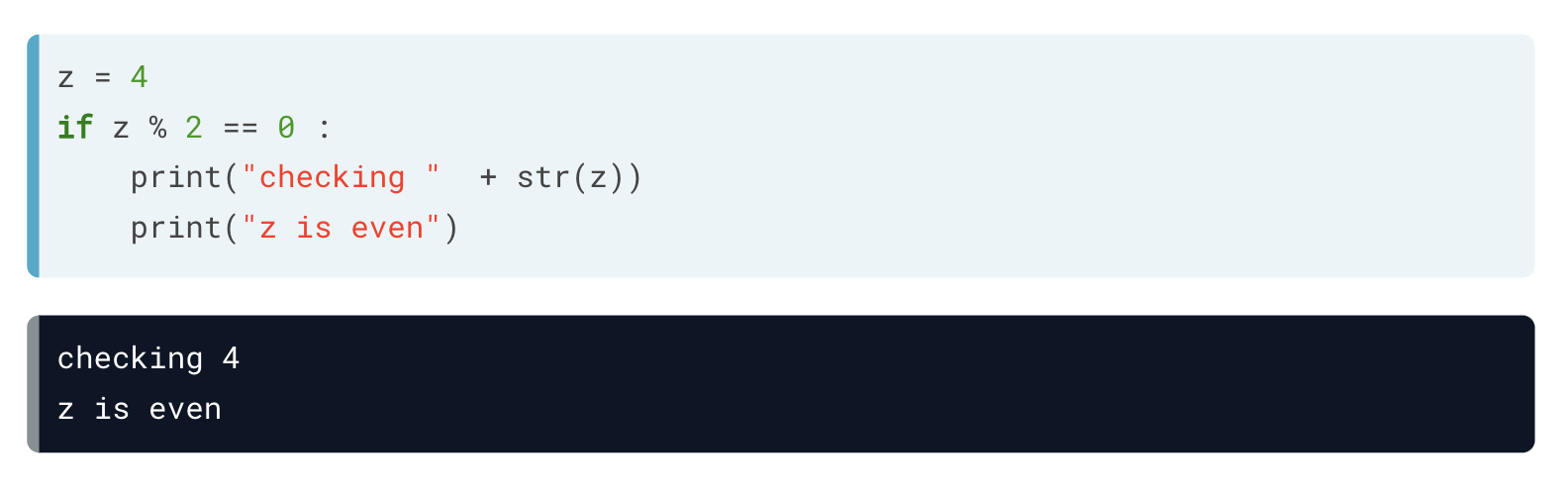##### Example of a False if statement

Let's change the value of z to be odd. You will notice that the code will not print anything since the condition will not be passed, i.e., False.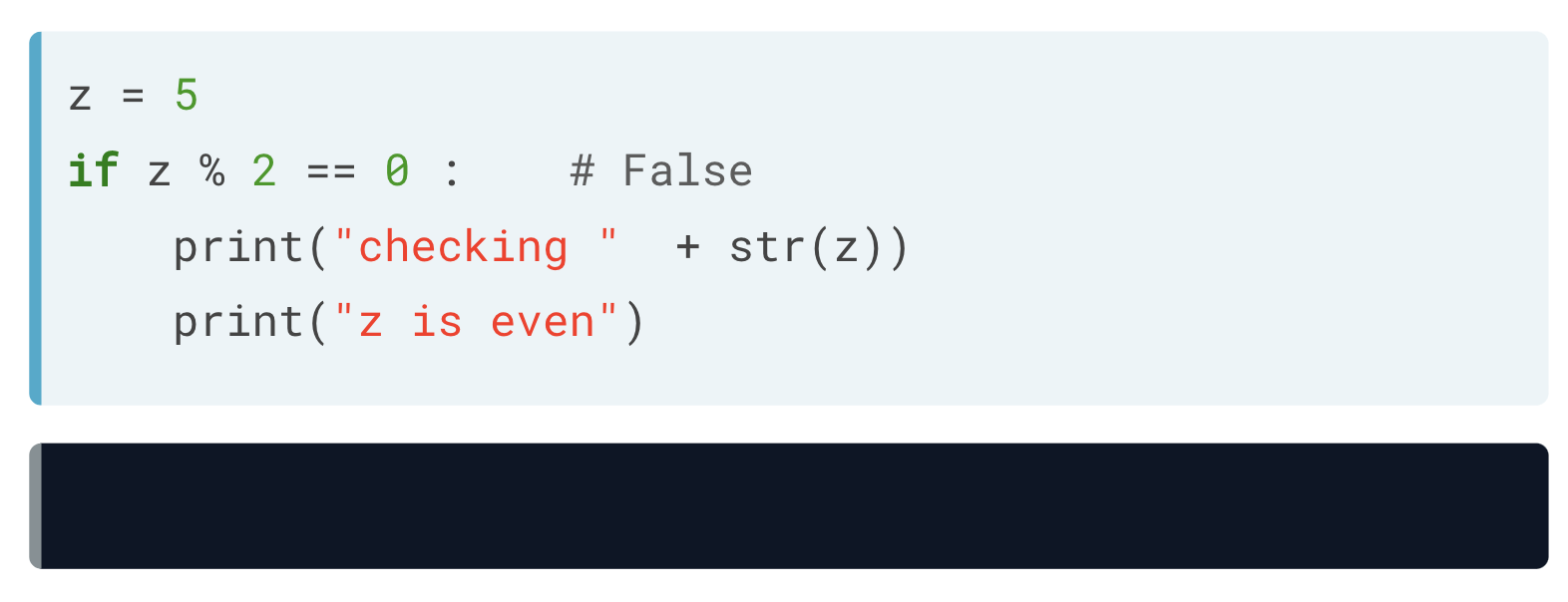## if-else condition

The if-else condition adds an additional step in the decision-making process compared to the simple if statement. The beginning of an if-else statement operates similar to a simple if statement; however, if the condition is false, instead of printing nothing, the indented expression under else will be printed.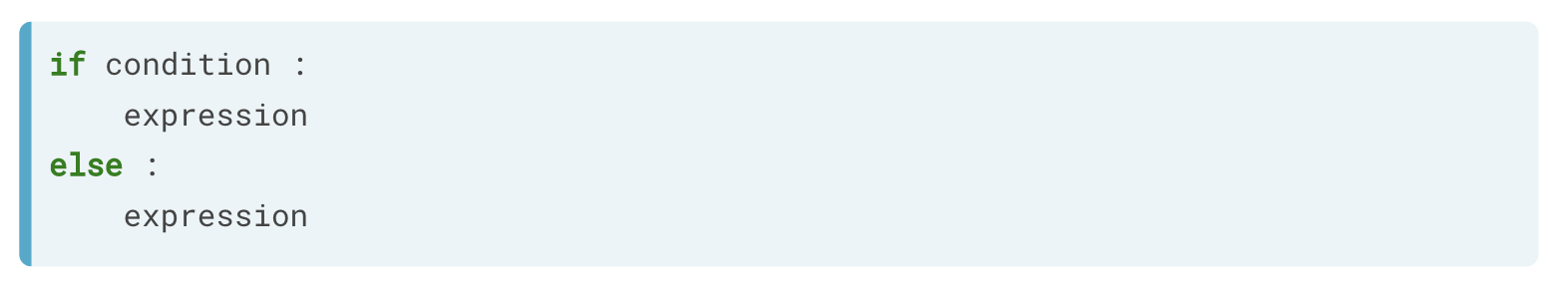### Example of if-else

Continuing our previous example, what if you want to print 'z is odd' when the if condition is false? In this case, you can simply add another condition, which is the else condition. If you run it with z equal to 5, the condition is not true, so the expression for the else statement gets printed out.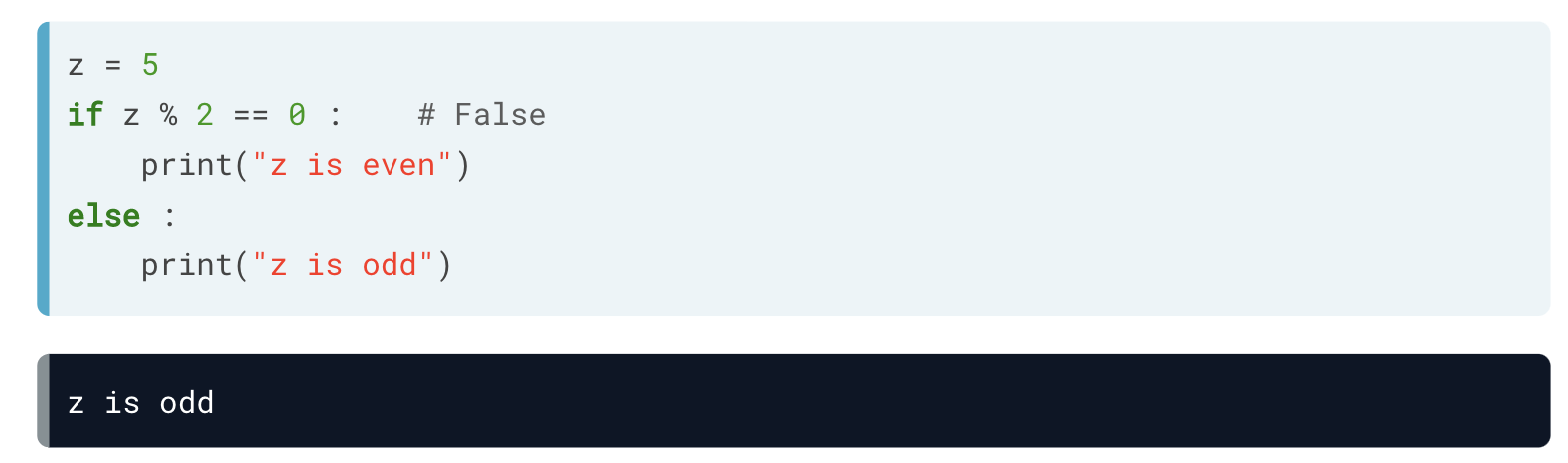## if-elif-else condition

The most complex of these conditions is the if-elif-else condition. When you run into a situation where you have several conditions, you can place as many elif conditions as necessary between the if condition and the else condition.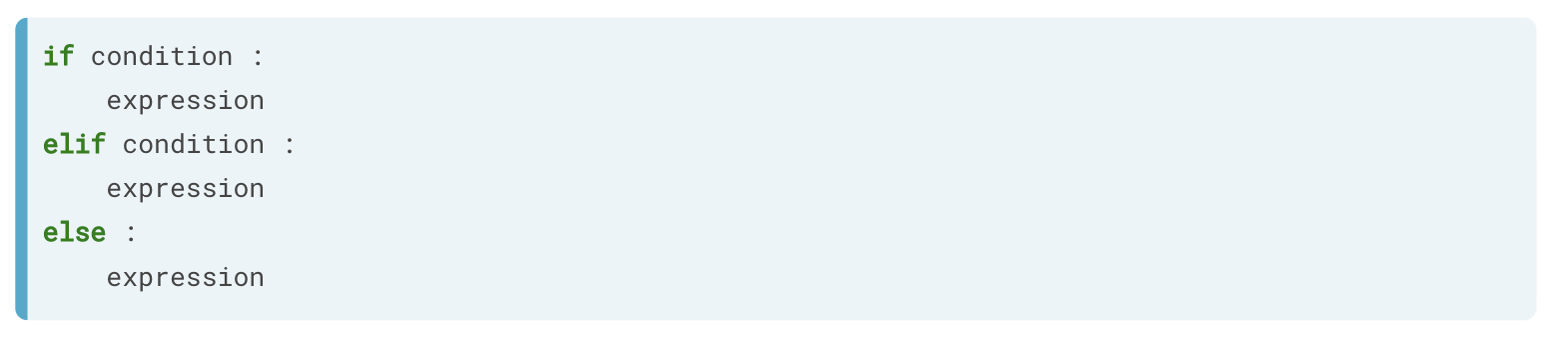### Example of if-elif-else condition

Below is an example of where you want different printouts for numbers that are divisible by 2 and 3.

Here, since z equals 3, the first condition is False, so it goes over to the next condition. The next condition does hold True. Hence, the corresponding print statement is executed.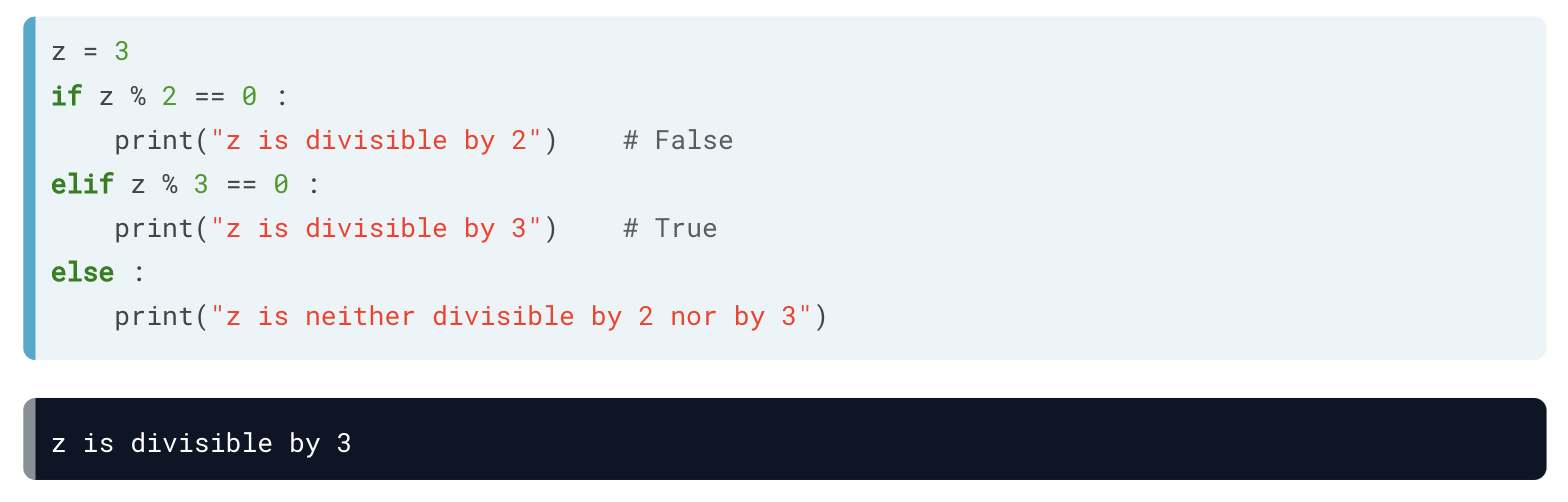### Example of if-elif-else condition

In the below example, you define two variables room and area. You then construct if-elif-else and if-else conditions each for room and area, respectively.

In the first condition, you check if you are looking in the kitchen, elif you are looking in the bedroom, else you are looking around elsewhere. Depending on the value of the room variable, the satisfied condition is executed.

Similarly, for the area variable, you write an if and else condition and check whether the area is greater than 15 or not.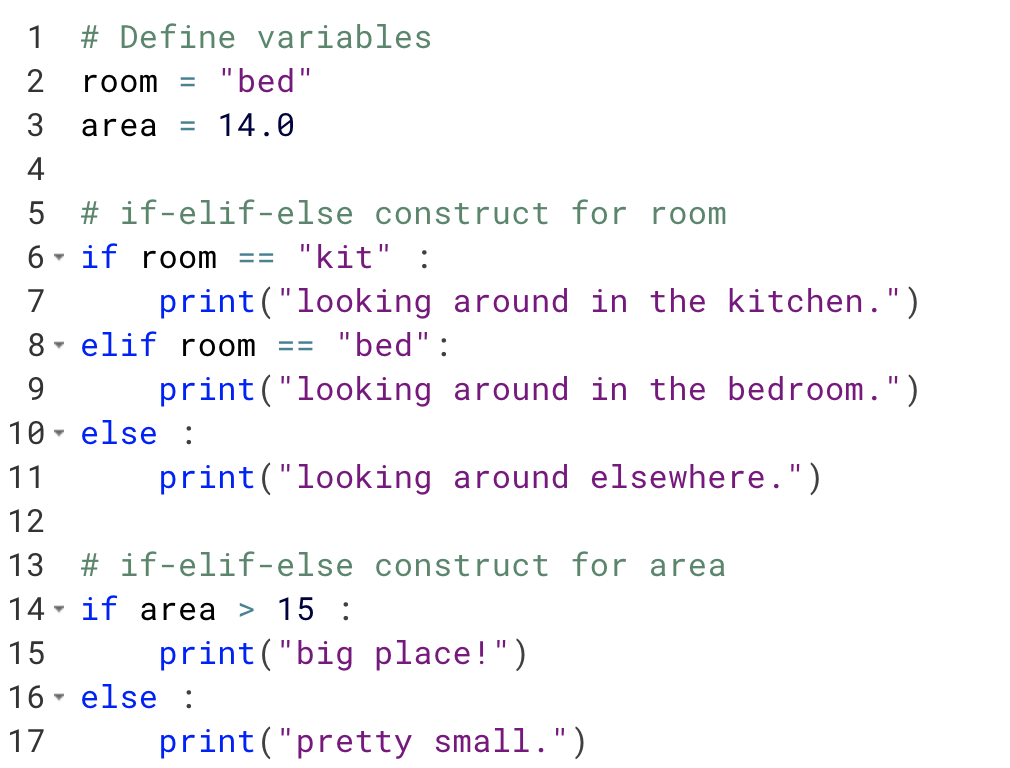When we run the above code, it produces the following result:

looking around in the bedroom. pretty small.